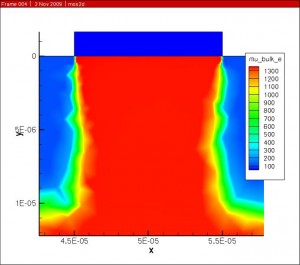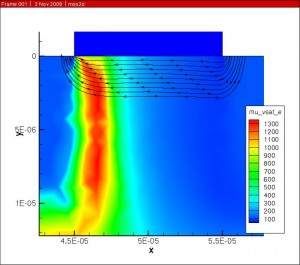# Category Archives: Announce

An interesting project from the IuE Summer of Code 2014 is a collection of 3D CAD models. On the CAD models page are examples of these devices.

• MOSFET and Single-Gate
• Double-Gate
• Tri Gate
• All-Around-Gate
• FlexFET
• Special Gate-Form Devices

Using the FreeCAD open source CAD modeling software. The student created these models from examples in the literature. There are also tutorials at the bottom of the page for building a Double-Gate MOSFET and Tri-Gate devices.

# 2dmatter.com

The NanoTCAD ViDES team has announced their new website, http://www.2dmatter.com.

From their announcement:

The website is intended to be a useful tool in order to link to all the recent publications concerned with Two-dimensional materials from the main journals in the field, i.e., (up to now) Physical Review Letters, Physical Review B, Applied Physics Letters, IEEE Transaction on Electron Devices, IEEE Electron Device Letters, IEEE Nanotechnology, Proceedings of IEEE, Scientific Reports, Nature Nanotechnology, ACS NANO and Nano Letters.

# Fujitsu Simulates Graphene Device Using Open Source Tool

Fujitsu announced the simulation of a 3030 Atom Nano device here on January 14th, 2013. The simulation took 20 hours on their supercomputer to simulate the electrical properties of graphene and an insulating layer, using OpenMX Material Explorer.

Even more interesting is the news that OpenMX is an open source tool available from openmx-square.org under the terms of the GPL.

# Welcome

This blog focuses on both EDA and TCAD software.  From time to time I’ll be posting about these topics and hope that you find these useful.

# DEVSIM is now Open Source

https://devsim.org

The direct link to the documentation is here:

https://devsim.org/manual

I hope that this software is useful to the TCAD simulation community. Please let me know if you have any questions about installation or usage of the software.

# Platform Support

DEVSIM is now supported on Mac OS X. The similarity to Linux and other Unix operating systems made this an easy process. DEVSIM now functions on the following platforms:

• Linux (32, 64 bit)
• Mac OS X (32, 64 bit)
• Windows (32 bit)
• # Mobility Modeling

Attached are pictures from a MOSFET simulation operating in the saturation region.

The simulation results are all for the same bias point using the combined electron mobility model.

The Klaassen (Philips) mobility model was implemented for doping and concentration dependence.The Darwish (Lucent) model was then used to account for the mobility dependence on electric field normal to current flow.The combined mobility was then scaled by the velocity saturation and is with respect to the electric field parallel to current flow to get the final result.Future work involves adapting the original Klaassen model so that it agrees with the modifications made in the Darwish paper.

The full implementation of the Klaassen model is presented here, and it tries to follow the original paper. The final mobility is placed on the edge connecting two nodes, and this is done using the geometric mean. Please let me know if you think there are any bugs in the implementation.

``` set mu_L_e "(mu_max_e * (300 / T)^theta1_e)" set mu_L_h "(mu_max_h * (300 / T)^theta1_h)" createNodeModel \$device \$region mu_L_e "\${mu_L_e}" createNodeModel \$device \$region mu_L_h "\${mu_L_h}" ```
``` set mu_e_N "(mu_max_e * mu_max_e / (mu_max_e - mu_min_e) * (T/300)^(3*alpha_1_e - 1.5))" set mu_h_N "(mu_max_h * mu_max_h / (mu_max_h - mu_min_h) * (T/300)^(3*alpha_1_h - 1.5))" createNodeModel \$device \$region mu_e_N "\${mu_e_N}" createNodeModel \$device \$region mu_h_N "\${mu_h_N}" ```
``` set mu_e_c "(mu_min_e * mu_max_e / (mu_max_e - mu_min_e)) * (300/T)^(0.5)" set mu_h_c "(mu_min_h * mu_max_h / (mu_max_h - mu_min_h)) * (300/T)^(0.5)" createNodeModel \$device \$region mu_e_c "\${mu_e_c}" createNodeModel \$device \$region mu_h_c "\${mu_h_c}" ```
``` set PBH_e "(1.36e20/(Electrons + Holes) * (m_e) * (T/300)^2)" set PBH_h "(1.36e20/(Electrons + Holes) * (m_h) * (T/300)^2)" createNodeModel \$device \$region PBH_e "\$PBH_e" createNodeModelDerivative \$device \$region PBH_e "\$PBH_e" Electrons Holes createNodeModel \$device \$region PBH_h "\$PBH_h" createNodeModelDerivative \$device \$region PBH_h "\$PBH_h" Electrons Holes ```
``` set Z_D "(1 + 1 / (c_D + (Nref_D / Donors)^2))" set Z_A "(1 + 1 / (c_A + (Nref_A / Acceptors)^2))" createNodeModel \$device \$region Z_D "\${Z_D}" createNodeModel \$device \$region Z_A "\${Z_A}" ```
``` set N_D "(Z_D * Donors)" set N_A "(Z_A * Acceptors)" createNodeModel \$device \$region N_D "\${N_D}" createNodeModel \$device \$region N_A "\${N_A}" ```
``` set N_e_sc "(N_D + N_A + Holes)" set N_h_sc "(N_A + N_D + Electrons)" createNodeModel \$device \$region N_e_sc "\$N_e_sc" createNodeModelDerivative \$device \$region N_e_sc "\$N_e_sc" Electrons Holes createNodeModel \$device \$region N_h_sc "\$N_h_sc" createNodeModelDerivative \$device \$region N_h_sc "\$N_h_sc" Electrons Holes ```
``` set PCW_e "(3.97e13 * (1/(Z_D^3 * N_e_sc) * (T/300)^3)^(2/3))" set PCW_h "(3.97e13 * (1/(Z_A^3 * N_h_sc) * (T/300)^3)^(2/3))" createNodeModel \$device \$region PCW_e "\$PCW_e" createNodeModelDerivative \$device \$region PCW_e "\$PCW_e" Electrons Holes createNodeModel \$device \$region PCW_h "\$PCW_h" createNodeModelDerivative \$device \$region PCW_h "\$PCW_h" Electrons Holes ```
``` set Pe "(1/(f_CW / PCW_e + f_BH/PBH_e))" set Ph "(1/(f_CW / PCW_h + f_BH/PBH_h))" createNodeModel \$device \$region Pe "\$Pe" createNodeModelDerivative \$device \$region Pe "\$Pe" Electrons Holes createNodeModel \$device \$region Ph "\$Ph" createNodeModelDerivative \$device \$region Ph "\$Ph" Electrons Holes ```
``` set G_Pe "(1 - s1 / (s2 + (1.0/m_e * T/300)^s4 * Pe)^s3 + s5/((m_e * 300/T)^s7*Pe)^s6)" set G_Ph "(1 - s1 / (s2 + (1.0/m_h * T/300)^s4 * Ph)^s3 + s5/((m_h * 300/T)^s7*Ph)^s6)" createNodeModel \$device \$region G_Pe "\$G_Pe" createNodeModelDerivative \$device \$region G_Pe "\$G_Pe" Electrons Holes createNodeModel \$device \$region G_Ph "\$G_Ph" createNodeModelDerivative \$device \$region G_Ph "\$G_Ph" Electrons Holes ```
``` set F_Pe "((r1 * Pe^r6 + r2 + r3 * m_e/m_h)/(Pe^r6 + r4 + r5 * m_e/m_h))" set F_Ph "((r1 * Ph^r6 + r2 + r3 * m_h/m_e)/(Ph^r6 + r4 + r5 * m_h/m_e))" createNodeModel \$device \$region F_Pe "\$F_Pe" createNodeModelDerivative \$device \$region F_Pe "\$F_Pe" Electrons Holes createNodeModel \$device \$region F_Ph "\$F_Ph" createNodeModelDerivative \$device \$region F_Ph "\$F_Ph" Electrons Holes ```
``` set N_e_sc_eff "(N_D + G_Pe * N_A + Holes / F_Pe)" set N_h_sc_eff "(N_A + G_Ph * N_D + Electrons / F_Ph)" createNodeModel \$device \$region N_e_sc_eff "\$N_e_sc_eff" createNodeModelDerivative \$device \$region N_e_sc_eff "\$N_e_sc_eff" Electrons Holes createNodeModel \$device \$region N_h_sc_eff "\$N_h_sc_eff" createNodeModelDerivative \$device \$region N_h_sc_eff "\$N_h_sc_eff" Electrons Holes ```
``` set mu_e_D_A_h "mu_e_N * N_e_sc/N_e_sc_eff * (Nref_1_e / N_e_sc)^alpha_1_e + mu_e_c * ((Electrons + Holes)/N_e_sc_eff)" set mu_h_D_A_e "mu_h_N * N_h_sc/N_h_sc_eff * (Nref_1_h / N_h_sc)^alpha_1_h + mu_h_c * ((Electrons + Holes)/N_h_sc_eff)" createNodeModel \$device \$region mu_e_D_A_h "\${mu_e_D_A_h}" createNodeModelDerivative \$device \$region mu_e_D_A_h "\${mu_e_D_A_h}" Electrons Holes createNodeModel \$device \$region mu_h_D_A_e "\${mu_h_D_A_e}" createNodeModelDerivative \$device \$region mu_h_D_A_e "\${mu_h_D_A_e}" Electrons Holes ```
``` set mu_bulk_e_Node "mu_e_D_A_h * mu_L_e / (mu_e_D_A_h + mu_L_e)" createNodeModel \$device \$region mu_bulk_e_Node "\${mu_bulk_e_Node}" createNodeModelDerivative \$device \$region mu_bulk_e_Node "\${mu_bulk_e_Node}" Electrons Holes ```
``` set mu_bulk_h_Node "mu_h_D_A_e * mu_L_h / (mu_h_D_A_e + mu_L_h)" createNodeModel \$device \$region mu_bulk_h_Node "\${mu_bulk_h_Node}" createNodeModelDerivative \$device \$region mu_bulk_h_Node "\${mu_bulk_h_Node}" Electrons Holes ```
``` createGeometricMean \$device \$region mu_bulk_e_Node mu_bulk_e createGeometricMeanDerivative \$device \$region mu_bulk_e_Node mu_bulk_e Electrons Holes createGeometricMean \$device \$region mu_bulk_h_Node mu_bulk_h createGeometricMeanDerivative \$device \$region mu_bulk_h_Node mu_bulk_h Electrons Holes ```

# Porting DEVSIM to Windows

While our primary development platform is 64-bit Linux, work is in process to get DEVSIM working on 32-bit Windows. It has been challenging, but thankfully the porting process has become easier as compiler vendors have become more standards compliant. It is especially nice that the majority of the third party components of our software already support Windows and Unix-like platforms.

One of the benefits of porting with a new compiler has been to identify code which is not necessarily portable across computer architectures, or entirely C++ standards compliant. This effort has even enabled us to find a few minor defects in our implementation.

I am happy to report that we have a functioning build on Windows, and thorough regression testing is in progress.